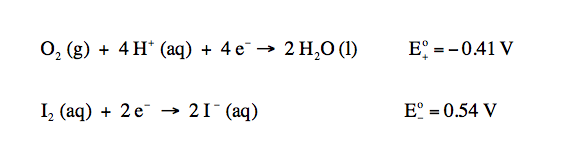Ch.16 - Electroanalytical TechniquesWorksheetSee all chapters
 Ch.1 - Chemical Measurements 2hrs & 10mins 0% complete Worksheet Ch.2 - Tools of the Trade 1hr & 26mins 0% complete Worksheet Ch.3 - Experimental Error 1hr & 51mins 0% complete Worksheet Ch.4 + 5 - Statistics, Quality Assurance and Calibration Methods 2hrs & 1min 0% complete Worksheet Ch.6 - Chemical Equilibrium 3hrs & 43mins 0% complete Worksheet Ch.7 - Activity and the Systematic Treatment of Equilibrium 1hr & 1min 0% complete Worksheet Ch.8 - Monoprotic Acid-Base Equilibria 1hr & 52mins 0% complete Worksheet Ch.9 - Polyprotic Acid-Base Equilibria 2hrs & 16mins 0% complete Worksheet Ch.10 - Acid-Base Titrations 2hrs & 22mins 0% complete Worksheet Ch.11 - EDTA Titrations 1hr & 37mins 0% complete Worksheet Ch.12 - Advanced Topics in Equilibrium 1hr & 18mins 0% complete Worksheet Ch.13 - Fundamentals of Electrochemistry 2hrs & 27mins 0% complete Worksheet Ch.14 - Electrodes and Potentiometry 55mins 0% complete Worksheet Ch.15 - Redox Titrations 1hr & 14mins 0% complete Worksheet Ch.16 - Electroanalytical Techniques 1hr & 23mins 0% complete Worksheet Ch.17 - Fundamentals of Spectrophotometry 55mins 0% complete Worksheet BONUS: Chemical Kinetics Not available yet

# Fundamentals of Electrolysis

See all sections
Sections
Fundamentals of Electrolysis
Gravimetric Analysis
Coulometry
Voltammetry
###### Electrolysis

Concept #1:

Electrolysis deals with passing an electrical current through a substance in order to produce chemical changes.

Example #1:

Aluminum can be electroplated at the cathode of an electrolysis cell by the half-reaction:

Al3+ (aq) + 3 e  → Al (s)

How much time would it take for 825 mg of aluminum to be plated at a current of 4.1 A?

Example #2: In the electrolysis of molecular iodine to iodide ions for 0.15 M NaI solution containing 4.2 x 10-4 M I2 at a pH = 6.00 with PO2 = 1.25 bar, calculate the voltage needed to drive the reaction.###### Electrolysis Calculations

Example #3:

Gold can be plated out of a solution containing Au3+ based on the following half reaction:

Au3+ (aq) + 3 e  → Au (s)

a) What mass of gold is plated by a 41 minute flow of 6.8 A current?

Example #4:

A solution of Mn+5 is used to plate out Mn in an electrochemical cell. If a total of 1.13 g of Mn is plated out in a total time of 1600 seconds, what was the electrical current used? (MW of Mn is 54.94 g/mol)

Example #5:

EXAMPLE: If steady current of 15 amperes is provided by a stable voltage of 12 Volts for 600 seconds, answer each of the following questions.

a)  Calculate the total charge that passes through the circuit in this time.

b)  Calculate the total number of moles of electrons that pass through the circuit in this time.

c)  Calculate the total amount of energy that passes through the circuit in this time.

d)  Calculate the power that the battery provides during this process.